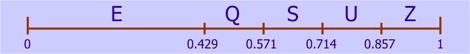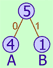# AIC - Context Adaptive Binary Arithmetic Coding

After a block has been predicted and DCT transformed, the resulting prediction modes and DCT coefficients must be stored in a stream. To minimize the number of bits needed to store these values, Advanced Image Coding uses the Context Adaptive Binary Arithmetic Coding (CABAC) algorithms from the H.264 standard. Below you will find a short introduction to arithmetic coding and the way it is used in H.264 and AIC. For more details on arithmetic coding, again refer to the Resources and links section.

The letters in CABAC will be discussed in reverse order:

## cabAC - Arithmetic Coding

Arithmetic coding is a statistical method for coding values that depends on the probabilities of these values. The most common method for statistical compression is Huffman coding.

For example, suppose we want to compress the word "SQUEEZE". This word contains 5 different symbols: E, Q, S, U and Z. To store this text without compression, you ideally need Log2(5) or 2.32 bits per symbol to store the word in 7 * 2.32 = 16.25 bits. However, since a computer can only handle complete bits, we need a minimum of 3 bits per symbol and store the word in 21 bits.

By using statistical modeling, we can assign less bits to common symbols (the E's in SQUEEZE) and more bits to uncommon symbols. According to Claude Shannon, the entropy — or information content — of a symbol depends on the probability of that symbol:

 Entropy = -Log2(Probability) or, Entropy = Log2(1/Probability)

The entropy is equal to the optimal number of bits to encode a symbol. The symbol E in SQUEEZE occurs three times and has a probability of 3/7, so the entropy of this symbol is around 1.22. The other symbols have a probability of 1/7 and an entropy of around 2.81. So the optimal number of bits to encode the word SQUEEZE is 3 * 1.22 + 4 * 2.81 = 14.90 bits.

When you encode this word using Huffman coding, you usually build a Huffman tree by recursively taking the two least common symbols and turning them into a tree node, and then repeat this process until one root node remains. Here is the Huffman tree for the word SQUEEZE:Each symbol is encoded by following the tree nodes from the root to the leaves, encoding a 0-bit when the left branch is taken, and a 1-bit when the right branch is taken. Thus, the symbols have the following codes:

 Symbol Bits Bit count Entropy E 0 1 1.22 Q 100 3 2.81 S 101 3 2.81 U 110 3 2.81 Z 111 3 2.81

The number of bits to encode the word SQUEEZE with Huffman coding is thus 3 * 1 + 4 * 3 = 15 bits, which is not so far from the optimal 14.90 bits.

The problem with Huffman coding is that you cannot encode a symbol in a fractional number of bits, which becomes a big problem when the alphabet has only 2 symbols, as we will see later. Arithmetic coding does not have this limitation and can achieve results much closer to the entropy or optimal number of bits. However, it is conceptually more difficult to understand than Huffman coding and usually slower as well (although a software implementation can be relatively simple).

With arithmetic coding, an entire word or message is coded as one single number in the range of 0 to 1 (not inclusive the 1). This range is first divided in to sub ranges, one for each symbol, where the length of each sub range is proportional to the probability of a symbol. In the SQUEEZE example, the E-range will thus have a length of 3/7 or 0.429, and Q, S, U and Z ranges will have a length of 1/7 or 0.143:Now we encode the first letter, S, which has the range 0.571 - 0.714. Again, we subdivide this range in 5 sub ranges:Next, we encode the letter Q, so we divide the range 0.633 - 0.653 in 5 sub ranges again. This process is repeated for the other letters:Eventually, the range for the last letter E becomes 0.64769 - 0.64772. This means that we can encode the word SQUEEZE with a single number in this range. The binary number in this range with the smallest number of bits is 0.101001011101, which corresponds to 0.647705 decimal. The '0.' prefix does not have to be transmitted because every arithmetic coded message starts with this prefix. So we only need to transmit the sequence 101001011101, which is only 12 bits. This number is even below the optimal number of bits of 14.90, but this is not entirely fair. Since the last letter of SQUEEZE is also the most common one, the final range is relatively large, making it easier to fit a value in it with less than the optimal number of bits. If the word was SQUEEEZ, we also would have needed 15 bits with arithmetic coding, but as messages get longer, arithmetic coding clearly outperforms Huffman coding.

The arithmetic decoding process is similar to the encoding process. We know we have transmitted the value 0.647705. Starting at the top of the figure above, we see that this number falls in the range 0.571 - 0.715, so the first letter must be an S. De decoder also subdivides this range, and we see that the value 0.647705 now falls in the range 0.633 - 0.653, so the second letter must be a Q. This process is repeated until the entire word SQUEEZE is decoded.

## caBac - Binary

H.264 and AIC use a form of arithmetic coding that only uses 2 symbols, 0 and 1; hence the word Binary Arithmetic Coding. Messages with just 2 symbols cannot be compressed with Huffman coding, because the tree would always look as follows, regardless of the probabilities of the symbols. The Huffman tree for the message "AABAA" would look like:I've renamed the symbols 0 and 1 to A and B to avoid confusion with the 0 and 1 bits in the Huffman codes. In the tree above, both A and B are represented with 1 bit, even if A had a probability of 99.99% and B a probability of 0.01%. So the message "AABAA" would be coded with 5 bits:

 Symbol Bits Bit count Entropy A 0 1 0.32 B 1 1 2.32

Ideally, A would be encoded with only 0.32 bits, and B with 2.32 bits, so the message AABAA can be encoded with 4 * 0.32 + 1 * 2.32 = 3.61 bits.

With arithmetic coding, AABAA can be represented with a number between 0.512 and 0.594. The binary number 0.1001 (0.5625) falls in this range and only takes 4 bits to transmit. For longer messages and skewed probabilities, the gains of binary arithmetic coding become even greater. In AIC and H.264, these probabilities are often skewed. For example, when encoding DCT coefficients, a 1-symbol is encoded for every coefficient that isn't 0. After prediction and DCT transformation, many coefficients are zero, and the probability of a coefficient being equal to 0 can become over 95%, especially when the coefficient context is considered (discussed later).

A disadvantage of binary arithmetic coding is that only two symbols, 0 and 1, can be encoded. Other symbols must first be binarised. For example, the prediction mode is binarised as follows. As discussed in the topic Block Prediction, the prediction mode itself is predicted from previous prediction modes. When the prediction is correct, the symbol 1 is encoded, otherwise the symbol 0. If the prediction is incorrect, the prediction mode is one of the other 8 available prediction modes, which can be represented using 3 bits. Each of these 3 bits is encoded as separate symbol.

In H.264 and AIC, all symbols are encoded in a context, and the probabilities of each symbol adapt to the context in which they are coded. Let's take the word SQUEEZE again for example. We could encode each letter with the previous letter as context, as in almost all languages, the probability of a letter depends on the previous letter in the text. An extreme example is the letter U in SQUEEZE. In English, this letter has an average probability of about 3%, but when the previous letter is a Q, the probability rises to almost 100%.

H.264 and AIC use several contexts. The probability of a DCT coefficient being 0 is greater for coefficients in the lower right corner of the 8x8 coefficient matrix as for coefficients in the upper left corner. In AIC, the position of the coefficient in the matrix is the context, so there are 64 contexts for encoding the probability of a coefficient being 0.

All contexts start out with probabilities that are appropriate for the chosen quality level. These initial probabilities are chosen after performing many tests on different images using different quality settings. As the image is being encoded or decoded, these probabilities adapt to the actual input values. It takes a little time for the probabilities to adapt to the image characteristics, and for very small images this can have an impact on the performance if the initial probabilities are not well chosen.

AIC uses the following contexts:

• Predicted prediction mode: one context for encoding if the predicted prediction mode is correct (1) or not (0).
• Prediction mode: if the predicted prediction mode is not correct, this context is used to encode the actual prediction mode.
• Coefficient map: contexts used to encode if a DCT coefficient is zero or not (in other words, if the coefficient is significant). One context is used for each position of the coefficient in the 8x8 matrix.
• Last coefficient: contexts for encoding if there are any more significant coefficients left. One context for each position in the matrix. When the previously encoded coefficient is the last significant coefficient in the block, this is encoded with a 1-symbol in this context. There is no need to encode any more coefficients for this block.
• Coefficient greater than 1: when a DCT coefficient is significant, there is a large probability that its value is only 1 or -1. This probability is encoded with this context.
• Absolute coefficient value: this context is only used when a coefficient is significant and not equal to 1 or -1. In that case the absolute value of the coefficient is binarised to a exponential Golomb code, which is then encoded with this context. The signs of the coefficients are not encoded within a context, because the probabilities of a coefficient being positive or negative are around 50%.
• Coded block: for lower quality levels, it frequently occurs that the entire block of coefficients is zero. This is coded with a single 0 or 1 symbol in this context.

## Implementation

The CABAC code in AIC is based on the code in the H.264 reference software. The binary arithmetic coding engine is implemented as a state machine, where probability estimation is performed by a transition process between 64 probability states for the Least Probable Symbol (LPS). The LPS is the least probable of the two symbols 0 or 1. The subdividing of the probability ranges is performed using look-up tables so there are no multiplications involved.